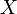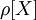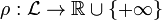## Options & Futures

Options and Futures represent two of the most common form of "Derivatives". Derivatives are financial instruments that derive their value from an 'underlying'. The underlying can be a stock issued by a company, a currency, Gold etc. more..

## Interest rates

An interest rate is the rate at which interest is paid by borrowers for the use of money that they borrow from a lender. Specifically, the interest rate (I/m) is a percent of principal (I) paid at some rate (m). more..

## Risk Measures

A risk measure is defined as a mapping from a set of random variables to the real numbers. This set of random variables represents the risk at hand. The common notation for a risk measure associated with a random variableis. A risk measureshould have certain properties. more..

## Stochastic Volatility

We have implemented some modules releted to stochastic volatility model. The modules are implemented from the research papers published in top financial conferences like “Bachelier Finance Society World Congress” in recent years. more..

## Computational Finance

Computational finance emphasizes practical numerical methods rather than mathematical proofs and focuses on techniques that apply directly to economic analyses. Applications of Computational Finance are Algorithmic Trading, Quant Trading, and High Performance Trading. more..

## Portfolio Optimization

Implemented module optimizes a portfolio under Mean-variance model. Given a return/price series of several assets, their expected return and expected covariance is estimated. Using these estimated values of the portfolio, mean-variance efficient frontier is calculated. more..